## 3.6 Globular clusters and satellites

Globular clusters and satellite galaxies are stellar components at large distances from the centre and are therefore ideal probes of the gravitational potential of a galaxy. The Milky Way may constitute, once more, the best example. Actually, globular clusters are typically at distances of less than 10 kpc, much lower than typical halo sizes, and are therefore not so suitable. Satellite galaxies are further away but they are statistically scarce, probably only nine satellites belonging to our Galaxy. However, satellites are also observable in other galaxies.

Suppose a Keplerian potential - GM/r and that, therefore, for satellites with elliptical orbits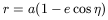(64)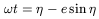(65)

as in 3.5.1. Time derivatives are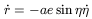(66)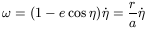(67)

Therefore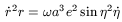(68)

Let us calculate the mean time value of this quantity during a complete orbit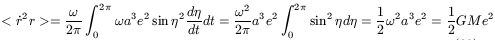(69)

Therefore, the galactic mass M could be obtained from the quantity <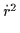r >. If we now consider not a single cluster or satellite but an assemblage of them in a given time, the mean time value would not differ from the mean value of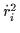ri of the different clusters, at present. We need to know the eccentricity, which would be different for the different clusters. The mean-square value for isotropic orbits is 1/2. Binney and Tremaine (1987), who have presented this argument, propose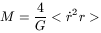(70)

This method was applied by Lynden-Bell et al. (1983), who obtained M = 3.8 × 1011M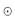with 9 satellites of the Milky Way (both Magellanic Clouds, Leo I and II, Fornax, Sculptor, Ursa Minor, Draco and Carina).

The adoption of a Keplerian potential was not fully justified as 6 out of the 9 selected satellites are at distances of less than 100 kpc, lower than a reasonable size of the dark matter halo. The unknown value of the eccentricities is also a major source of errors. If the orbits were radially elongated, with e = 1, the calculated mass would be closer to the galactic mass deduced from the visible matter alone. That orbits could be elongated rather than isotropic is somewhat suggested by the fact that (with the exceptions of Leo I and Leo II, which are too far away) all the satellites of the Milky Way are roughly aligned in a line connecting ( b = - 45o, l = 270o)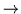(b = 45o, l = 90o).

Ashman (1992) and Trimble (1987) have summarized previous work carried out by Little and Tremaine (1991), Zaritsky et al. (1989), Salucci and Frenk (1989), Peterson and Latham (1989), Kulessa and Lynden-Bell (1992) and others. The results obtained by the different authors are very different, depending on the inclusion or exclusion of some distant satellites, in particular on the inclusion or exclusion of Leo I. However, a mass of 1012Mand a halo radius of 100 kpc are typical values.

This type of analysis will be more promising in the future, when proper motions of the satellites of the Milky Way become available. Wilkinson and Evans (1999) have incorporated into the computation the known proper motions of 6 satellites. They obtain a value of about 2 × 1012Mfor the mass of the Milky Way, with the inclusion or exclusion of Leo I not being so important as when only radial motions are considered. The results are model dependent and these authors have chosen a peculiar one, called the "truncated flat" rotation curve, in which the density decreases as r-2 in the inner parts and decreases as r-5 in the outer ones.

Tidal radii (see Section 3.3) of globular clusters and satellite galaxies have also been considered (e.g. Innanen et al. 1983) but these radii are obtained by an extrapolation of the photometric data, which introduces a lot of uncertainty. A mass of about 9 × 1011Mand a halo size of at least 44 kpc were obtained by these authors.

Satellites of other galaxies have been studied by Zaritsky et al. (1993), Zaritsky and White (1994), Zaritsky (1997) and others. The problem has much in common with that of binary galaxies but presents particular interest because, as satellites are supposed to be low mass systems they can be considered as test particles orbiting in the total mass of the primary. The above authors have observed 115 satellites around 69 isolated primary galaxies. They conclude that DM halos do exist and that they extend to distances of over 400 kpc, actually a very large figure, but many characteristic facts are difficult to explain: a) There is a complete lack of correlation between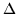v andr, which impedes the obtention of the mean value of galaxies. b) There is a complete lack of correlation betweenv and the HI widths. It has been mentioned that Salucci and Persic (1997) and, as will be mentioned later, theoretical models do not expect a large correlation between these two quantities, but not a vanishing one, either. c) Satellites seem to be preferentially distributed near the plane perpendicular to the rotation axis of the primary. d) The assemblage of all the satellites seems to present a "rotation curve" around a typical primary without signs of becoming flat. Note that the possibility of a common DM halo without an internal DM structure is a picture compatible with these observations.